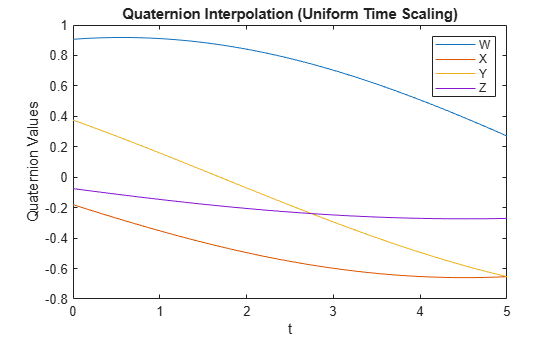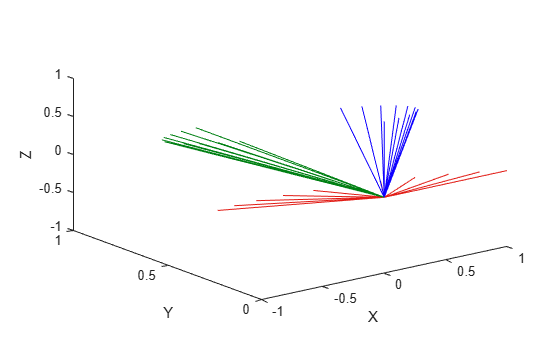# rottraj

Generate trajectories between orientation rotation matrices

Since R2019a

## Syntax

``[R,omega,alpha] = rottraj(r0,rF,tInterval,tSamples)``
``[R,omega,alpha] = rottraj(r0,rF,tInterval,tSamples,Name=Value)``

## Description

example

````[R,omega,alpha] = rottraj(r0,rF,tInterval,tSamples)` generates a trajectory that interpolates between two orientations, `r0` and `rF`, with points based on the time interval and given time samples.```
````[R,omega,alpha] = rottraj(r0,rF,tInterval,tSamples,Name=Value)` specifies additional parameters using name-value arguments.```

## Examples

collapse all

Define two quaternion waypoints to interpolate between.

```q0 = quaternion([0 pi/4 -pi/8],'euler','ZYX','point'); qF = quaternion([3*pi/2 0 -3*pi/4],'euler','ZYX','point');```

Specify a vector of times to sample the quaternion trajectory.

`tvec = 0:0.01:5;`

Generate the trajectory. Plot the results.

```[qInterp1,w1,a1] = rottraj(q0,qF,[0 5],tvec); plot(tvec,compact(qInterp1)) title('Quaternion Interpolation (Uniform Time Scaling)') xlabel('t') ylabel('Quaternion Values') legend('W','X','Y','Z')```Define two rotation matrix waypoints to interpolate between.

```r0 = [1 0 0; 0 1 0; 0 0 1]; rF = [0 0 1; 1 0 0; 0 0 0];```

Specify a vector of times to sample the quaternion trajectory.

`tvec = 0:0.1:1;`

Generate the trajectory. Plot the results using `plotTransforms`. Convert the rotation matrices to quaternions and specify zero translation. The figure shows all the intermediate rotations of the coordinate frame.

```[rInterp1,w1,a1] = rottraj(r0,rF,[0 1],tvec); rotations = rotm2quat(rInterp1); zeroVect = zeros(length(rotations),1); translations = [zeroVect,zeroVect,zeroVect]; plotTransforms(translations,rotations) xlabel('X') ylabel('Y') zlabel('Z')```## Input Arguments

collapse all

Initial orientation, specified as a 3-by-3 rotation matrix, a scalar `quaternion` object, or a scalar `so3` object. The function generates a trajectory that starts at the initial orientation, `r0`, and goes to the final orientation, `rF`.

`r0` and `rF` must be of the same type. For example, if `r0` is a scalar `so3` object, then `rF` must be a scalar `so3` object.

Example: ```quaternion([0 pi/4 -pi/8],"euler","ZYX","point");```

Data Types: `single` | `double`

Final orientation, specified as a 3-by-3 rotation matrix, a scalar `quaternion` object, or a scalar `so3` object. The function generates a trajectory that starts at the initial orientation, `r0`, and goes to the final orientation, `rF`.

`r0` and `rF` must be of the same type. For example, if `r0` is a scalar `so3` object, then `rF` must be a scalar `so3` object.

Example: ```quaternion([3*pi/2 0 -3*pi/4],"euler","ZYX","point")```

Data Types: `single` | `double`

Start and end times for the trajectory, specified as a two-element vector.

Example: `[0 10]`

Data Types: `single` | `double`

Time samples for the trajectory, specified as an m-element vector.

Example: `0:0.01:10`

Data Types: `single` | `double`

### Name-Value Arguments

Specify optional pairs of arguments as `Name1=Value1,...,NameN=ValueN`, where `Name` is the argument name and `Value` is the corresponding value. Name-value arguments must appear after other arguments, but the order of the pairs does not matter.

Before R2021a, use commas to separate each name and value, and enclose `Name` in quotes.

Example: `TimeScaling=[0 1 2; 0 1 0; 0 0 0]`

Time scaling vector and the first two derivatives, specified as the comma-separated pair of `TimeScaling` and a 3-by-m vector, where m is the length of `tSamples`. By default, the time scaling is a linear time scaling between the time points in `tInterval`.

For a nonlinear time scaling, specify the values of the time points in the first row. The second and third rows are the velocity and acceleration of the time points, respectively. For example, to follow the path with a linear velocity to the halfway point, and then jump to the end, the time-scaling would be:

```s(1,:) = [0 0.25 0.5 1 1 1] % Position s(2,:) = [1 1 1 0 0 0] % Velocity s(3,:) = [0 0 0 0 0 0] % Acceleration```

Data Types: `single` | `double`

## Output Arguments

collapse all

Orientation trajectory, returned as a 3-by-3-by-m rotation matrix array, an m-element array of `quaternion` objects, or an m-element array of `so3` objects. m is the number of points in `tSamples`. The output type matches the input type of `r0` and `rF`.

Orientation angular velocity, returned as a 3-by-m matrix, where m is the number of points in `tSamples`.

Orientation angular acceleration, returned as a 3-by-m matrix, where m is the number of points in `tSamples`

## Limitations

• When specifying your `r0` and `rF` input arguments as a 3-by-3 rotation matrix, they are converted to a `quaternion` object before interpolating the trajectory. If your rotation matrix does not follow a right-handed coordinate system or does not have a direct conversion to quaternions, this conversion may result in different initial and final rotations in the output trajectory.

 Dam, Erik B., Martin Koch, and Martin Lillholm. Quaternions, Interpolation and Animation. Technical Report DIKU-TR-98/5 (July 1998). http://web.mit.edu/2.998/www/QuaternionReport1.pdf

 Graf, Basile. Quaternions and Dynamics. arXiv:0811.2889 [math.DS] (2008). https://arxiv.org/pdf/0811.2889.pdf

 Lynch, Kevin M., and Frank C. Park. Modern Robotics: Mechanics, Planning, and Control. Cambridge University Press, 2017.